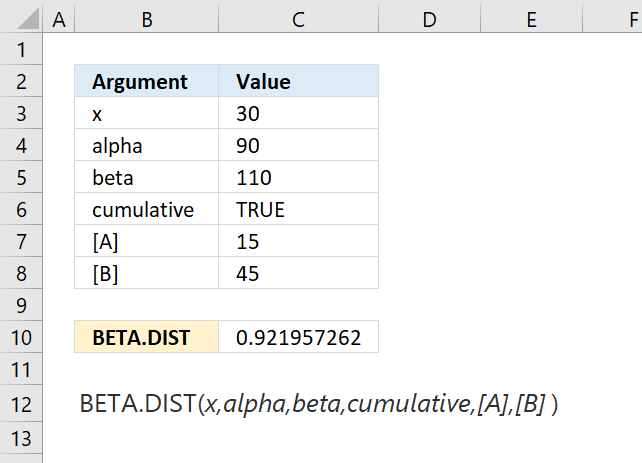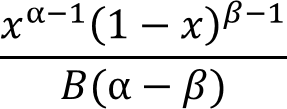Author: Oscar Cronquist Article last updated on October 01, 2018The BETA.DIST function calculates the beta distribution. It represents outcomes in the form of probabilities. This function was introduced in Excel 2010.

Formula in cell C10:

=BETA.DIST(C3,C4,C5,C6,C7,C8)

### Excel Function Syntax

BETA.DIST(x,alpha,beta,cumulative,[A],[B])

### Arguments

 x Required. alpha Required. A parameter which determines the shape of the distribution. beta Required. A parameter which determines the shape of the distribution. cumulative Required. A boolean value that determines the form of the function. TRUE - Cumulative beta probability distribution function FALSE - Beta probability density function [A] Optional. Lower bound, default value 0 (zero). [B] Optional. Upper bound, default value 1.